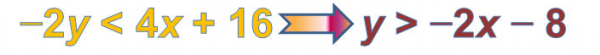# Let's Get Started

You will explore how to graph inequalities in order to solve.  You will also solve inequalities using properties of equality.  `

TEKS Standards and Student Expectations

A(3) Linear functions, equations, and inequalities. The student applies the mathematical process standards when using graphs of linear functions, key features, and related transformations to represent in multiple ways and solve, with and without technology, equations, inequalities, and systems of equations. The student is expected to:

A(3)(D) graph the solution set of linear inequalities in two variables on the coordinate plane

A(5) Linear functions, equations, and inequalities. The student applies the mathematical process standards to solve, with and without technology, linear equations and evaluate the reasonableness of their solutions. The student is expected to:

A(5)(B) solve linear inequalities in one variable, including those for which the application of the distributive property is necessary and for which variables are included on both sides

Resource Objective(s)

The student will represent linear inequalities using equations, tables, and graphs. The student will solve linear inequalities using graphs or properties of equality, and determine whether or not a given point is a solution to a linear inequality.

Essential Questions

How do you know when to use a solid or dashed line when graphing an inequality?

How do you know if you should shade above or below the line when graphing an inequality?

What are the similarities and differences in graphing an equation in slope-intercept form, and an inequality in slope-intercept form?

Vocabulary

# Introduction

You may have previously investigated different ways to determine if a given ordered pair was a solution to a linear inequality.

Is (-1, -7) a solution for 4x − 2y > 8?

 Use Substitution If we substitute -1 for x and -7 for y, we will have the following:   4x − 2y > 8 4(-1) − 2(-7) > 8 -4 + 14 > 8 10 > 8 Since 10 is greater than 8, we know that (-1, -6) is a solution to the inequality. Make a table of values Step 1: Enter the inequality in the function editor (Y=). Remember you may need to write the inequality in Y = form, or solve the inequality for y.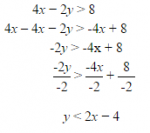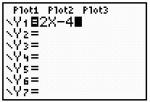. Step 2: Look at the table of values. Scroll to locate the x-value of -1.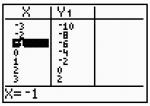The table of values shows that when x = -1, y = -6. Our test point is (-1, -7). Compare the y-value from the table with the y-value from the test point. The y-value from test point is less than the  y-value from table since -7 Since the y-value from the test point is less than the y-value from the table, the test point makes the inequality true. So, we know that (-1, -7) is a solution to the inequality. Use a graph Step 1: Enter the inequality in the function editor (Y=). Remember you may need to write the inequality in Y = form, or solve the inequality for y.We want to see y-values that are less than the expression 2x – 4, so set the graph to shade beneath the graph. Step 2: Look at the graph below. Locate the test point, (-1, -7). Since the test point lies within the shaded region, we know that (-1, -7) is a solution to the inequality.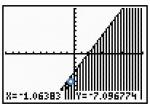You will look more closely at solving linear inequalities using two methods: graphs and properties of equality. You will also use those methods to solve linear inequalities that represent real-world situations.

# Solving Linear Inequalities Using Graphs

As with linear equations, linear inequalities can be represented in two forms.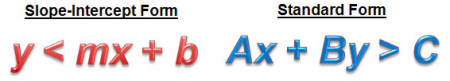You can graph a linear inequality from either form, just as you can with linear equations. However, since an inequality has multiple solution possibilities, there are additional steps that need to be taken to completely graph a linear inequality.

Use this inequality grapher applet to complete the table below in your notes. For directions on how to use it, view the following image, and click on the directions tab in the top left corner of the applet.

After completing the table, you can check your answer by viewing the next slide.  Use the information contained in the table to answer the questions that follow.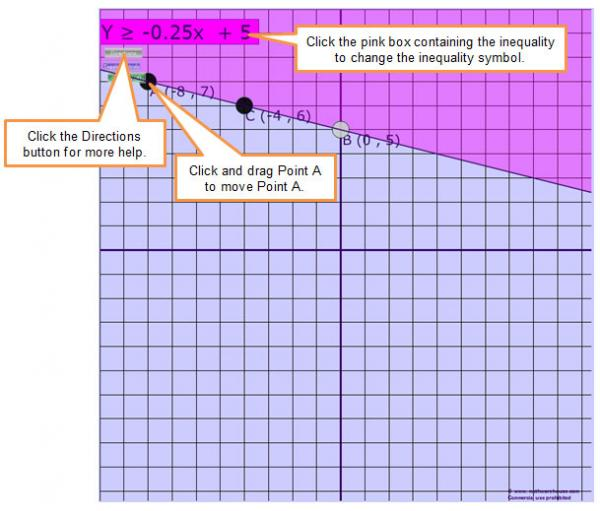Pause and Reflect

Click on each question to see the answer.

You can also graph inequalities on a graphing calculator. View this link to learn more about how to graph inequalities on a graphing calculator.

Practice

Graph each of the following inequalities.  Then drag the inequality to match its corresponding graph.

# Solving Linear Inequalities Using Properties of Equality

Now you will focus on using properties of equality to solve linear inequalities that come from problem situations. Don't forget, the inequality sign reverses if you multiply or divide by a negative number when solving an inequality for y.

Example 1

Carla and Crystal own a coffee shop. They want to create a house blend of coffee from two beans. Costa Rica Sunrise beans cost $7.50 per pound, and Colombia’s Best beans cost$8.75 per pound. They want to create a blend of beans that costs no more than $120 per batch. Carla has suggested that they use the following inequality, where x represents the number of pounds of Costa Rica Sunrise beans, and y represents the number of pounds of Colombia’s Best beans. 7.50x + 8.75y ≤ 120 Example 2 Hope and Todd run a small business producing snack boxes. Almonds cost$8.25 per pound, and cashews cost $12.50 per pound. They want to keep the cost of one batch of snack mix below$225. Hope wrote the inequality 8.25x + 12.50y < 225, where x represents the number of pounds of almonds, and y represents the number of pounds of cashews in a batch of snack mix.  Click on the slides below for hints and answers to the following questions.

# Summary

In this lesson you extended what you know about solving one-variable inequalities and linear equations to solving linear inequalities.

Linear inequalities may be solved graphically or symbolically using the properties of equality. In a graph, the solution set for an inequality is represented in the coordinate plane using two features: a boundary line and a shaded region.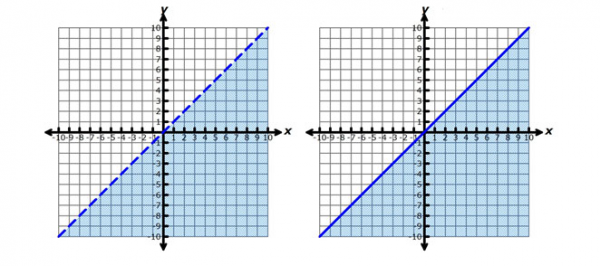If the boundary line is dashed, then the points along the boundary line are not included in the solution set of the inequality. If the boundary line is solid, then the points along the boundary line are included in the solution set of the inequality.

Properties of equality can also be used to solve linear inequalities. Solving linear inequalities is an algebraic process that is very similar to solving linear equations, with one additional feature. If multiplication or division by a negative number is used, the inequality symbol must be reversed in order to preserve the value of the inequality.